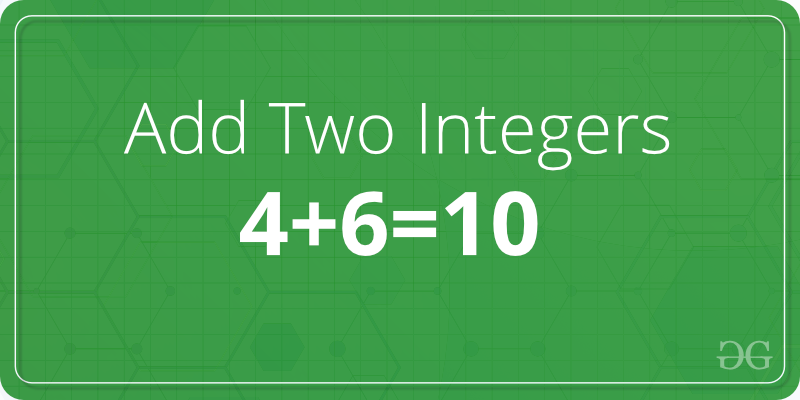Related Articles

# C Program to Add two Integers

• Difficulty Level : Basic
• Last Updated : 02 Nov, 2018

Given two numbers A and B. The task is to write a program to find the addition of these two numbers.Examples:

```Input: A = 2, B = 3
Output: 5

Input: A = 3, B = 6
Output: 9
```

## Recommended: Please try your approach on {IDE} first, before moving on to the solution.

In the below program to add two numbers, the user is first asked to enter two numbers and the input is scanned using the scanf() function and stored in the variablesand. Then, the variablesandare added using the arithmetic operatorand the result is stored in the variable sum.

Below is the C program to add two numbers:

 `// C program to add two numbers``#include`` ` `int` `main()``{``    ``int` `A, B, sum = 0;``     ` `    ``// Ask user to enter the two numbers``    ``printf``(``"Enter two numbers A and B : \n"``);``     ` `    ``// Read two numbers from the user || A = 2, B = 3``    ``scanf``(``"%d%d"``, &A, &B);``     ` `    ``// Calclulate the addition of A and B``    ``// using '+' operator``    ``sum = A + B;``     ` `    ``// Print the sum``    ``printf``(``"Sum of A and B is: %d"``, sum);``     ` `    ``return` `0;``}`

Output:

```Enter two numbers A and B : 2 3
Sum of A and B is: 5
```

Want to learn from the best curated videos and practice problems, check out the C Foundation Course for Basic to Advanced C.

My Personal Notes arrow_drop_up